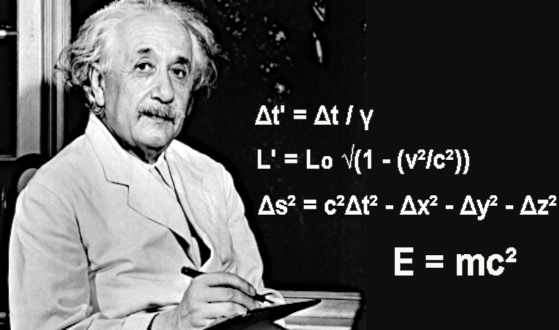Category: matter and particles
Updated 03 August 2023

# Special relativity equations (1905)Image: Albert Einstein (1879-1955) is a theoretical physicist, Nobel Prize in Physics (1921) on the corpuscular nature of light, through the study of the photoelectric effect.

## The postulates of special relativity

Special relativity is a physical theory formulated by Albert Einstein in 1905. It deals with the behavior of objects and physical phenomena in the presence of high speeds, close to the speed of light in vacuum (299,792,458 m/s).

Special relativity is based on two postulates:
- The principle of relativity: The laws of physics are the same for all inertial observers (observers in uniform motion relative to each other). There is no preferred repository.
- The constancy of the speed of light: The speed of light in vacuum is a universal constant, independent of the movement of the light source and the observer. This speed is always equal to approximately 299,792,458 m/s, or "c" in the equations of Special Relativity.

## The main consequences of Special Relativity

• Time dilation, also called time dilation, is one of the key effects of special relativity. Moving clocks relative to a resting observer seem to slow down. Time passes more slowly for a fast moving object compared to a "still" observer.
The effect of proper time is expressed in the formula: Δt' = Δt / γ
- Δt' is the time interval measured by a fast moving observer
- Δt is the time interval measured by an observer at rest
- γ (gamma) is the Lorentz factor defined as γ = 1 / √(1 - (v²/c²)), where "v" is the relative speed of the object and "c" is the speed of light in the void.

• Length contraction, also known as Lorentz contraction, is a phenomenon predicted by the special theory of relativity.
When an object is moving at a high speed relative to an observer at rest, that observer will see the object contracted in the direction of its motion. Fast-moving objects appear to shorten in the direction of their motion, when viewed by an observer at rest.
The Lorentz contraction for length is given by the formula: L' = L₀ * √(1 - (v²/c²))
- L' is the length measured by a fast moving observer
- L₀ is the proper length (length measured by an observer at rest)
- v is the relative speed of the object
- c is the speed of light in vacuum.

• The invariance of the space-time interval is a fundamental concept of Albert Einstein's special relativity. It expresses the fact that the space-time interval between two events, whatever the inertial reference chosen to observe them, remains invariant, ie it has the same value for all observers. The space-time interval between two events is a combination of the space and time intervals separating these events in a given reference frame. In special relativity, a specific metric is used to define the space-time interval, denoted Δs². In other words, space-time interval invariance means that if two observers move at constant and different speeds relative to each other, they will measure different intervals of time and space separating the same events. However, the quantity Δs², which combines these intervals of space and time, will be the same for all observers.
The invariance of the space-time interval is expressed in the formula: Δs² = c²Δt² - Δx² - Δy² - Δz²
- Δs² is the space-time interval
- c is the speed of light in vacuum
- Δt is the time interval between the two events
- Δx, Δy and Δz are the space intervals in the three dimensions.

• Mass-energy equivalence is a consequence of special relativity. It means that the mass of an object is a form of manifestation of its energy, and conversely, that energy can be converted into mass. In other words, mass and energy are actually two aspects of the same physical quantity. The mass-energy equivalence is expressed in the famous formula E=mc².
- E is the energy of an object
- m is its mass
- c is the speed of light in vacuum.

## E=mc²

E=mc2 is the best known and popularized equation in all of physics. It appeared in 1912 in a note by Albert Einstein, 7 years after the article of June 30, 1905 entitled "On the electrodynamics of bodies in motion". This 1905 article contains the basis of what will later be called "The Special Theory of Relativity".
E=mc² was one of the most revolutionary discoveries in modern physics and had a significant impact on our understanding of the universe.
The Special Relativity equations show how time, space and energy are affected by rapidly moving objects.
Special Relativity has been experimentally confirmed time and time again and has proven to be a solid and accurate theory for describing the behavior of fast moving objects at speeds close to the speed of light. It is an essential pillar of modern physics and paved the way for the more general theory of general relativity.

1997 © Astronoo.com − Astronomy, Astrophysics, Evolution and Earth science.